Study Guide

## Charge

The building block of all theories of electricity is charge. Charge is a fundamental property of matter, just like mass. Like mass describes the interaction of matter and gravitational fields, charge describes the interaction of matter and electric fields. Mass leads to all of the concepts in mechanics, from forces to energy to momentum, while charge will lead the charge into all of the concepts in electromagnetism. (Pun intended. We apologize.)

Charge is denoted q and is measured in coulombs (C). The coulomb is the basic SI unit of electromagnetism, just like the kilogram is the basic unit of mass.

Charge has three central properties, some of which are shared with mass and some of which are new:

• Polarity: Charge comes in two varieties, which we call positive and negative. Like charges (two positive or two negative) will repel, trying to push away from each other, while opposite charges (one negative and one positive) will attract, pulling each other closer. The phrase "opposites attract" has to come from somewhere other than Paula Abdul, right?
• Conservation of charge: Charge cannot be created or destroyed, only moved from one object to another or masked by charges of the opposite polarity. This is completely analogous to conservation of mass.
• Quantization of charge: Charge comes in discrete little packets equal to the magnitude of the charge of an electron (sometimes called the elementary charge, or e): 1.6 × 10-19 C. This is because all charge in the universe comes from electrons and protons, which have exactly equal and opposite charges, and there's no such thing as a half proton or quarter electron to add to the mix.* At the most basic level, mass is quantized, too, coming in units equal to the mass of protons and neutrons and electrons, but quantization of charge is much more evident in everyday life.

### Coulomb's Law

The interaction of positive and negative charges is given by Coulomb's Law. For two charges, q1 and q2, a distance r apart from each other, the force one exerts on the other (remember Newton's Third Law) is given by: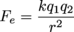The k in this equation is a fundamental constant often called Coulomb's constant, equal to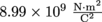.Note how similar this is to the equation for gravitational attraction between two masses: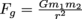. Both electric and gravitational forces are "r-squared" (sometimes called "inverse square") laws that describe the attractive or repulsive interactions of objects that do not touch. The interaction gets much, much larger as the objects get closer.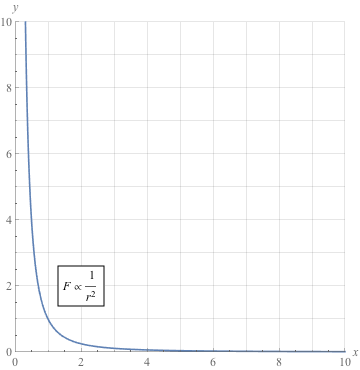Two like charges (plus-plus or minus-minus) will yield a positive Fe; this is the sign that represents a repulsive force. Two opposite charges (plus-minus) will make Fe negative, which is an attractive force (hubba hubba). If we think of force as something that pushes, then it makes sense that a positive force would push the particles apart and a negative force would bring them together.

That's all well and good for two charges, but there are more than just two charges floating around in the universe. Darn. Never fear, however, because charge obeys the principle of superposition. Superposition is a mathematical tool that lets us ignore everything in a problem except for one item, solve the problem, and then keep chugging away—ignore everything but the next item, solve, ignore everything but the item after that, solve, and so on. Ignore all those extra charges like you would a yappy beagle outside your window. Go ahead. At the end, we add all the little solutions together and that's our net answer.

For example, imagine a positive charge sitting between two negative charges.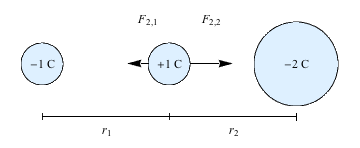The negative charge on the left pulls the positive charge left, with a force given by Coulomb's Law. But at the same time, the negative charge on the right pulls the positive charge right. The positive charge is in a game of electric tug-of-war, so that the net force it feels is the sum of these two forces, and will depend on the size of the negative charges and the distance between them and the positive charge.

### Common Mistakes

There are two directions at work in Coulomb forces. One is the sign that results from Coulomb's Law—whether the force is attractive or repulsive. But the other is what direction "attraction" or "repulsion" actually acts in, which is completely dependent on the geometry of the charge configuration. Attraction and repulsion will both always act along the line between the two charges, with attraction pulling them together and repulsion pushing them apart.

### Brain Snack

One of the most impressive ways to build up a huge chunk of static electricity, that thing your socks get in the dryer or that helps with bad hair days, is a van de Graaff generator.

*If you've heard of quarks, shush. They're never found outside of protons or neutrons anyway.